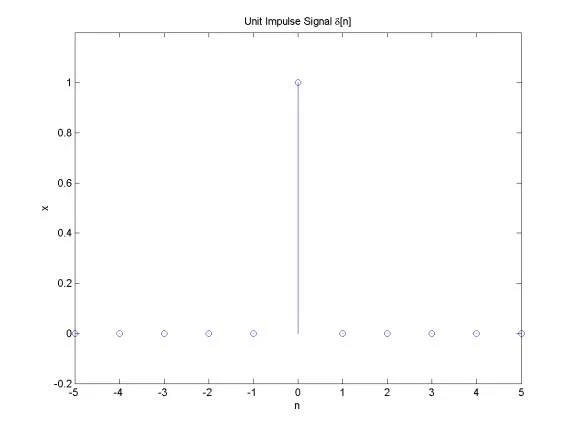## Digital system equationBack to DSP thread. Lets talk about digital system equation. Every digital system can be described using this equation. The output of digital filter generally consist of previous inputs and previous outputs. y(n-k) is considered as previous outputs; x(n-p) – previous inputs; ak and b­p – coefficients; This equation is convenient to define discrete system and extract various characteristics. Number N defines discrete filter tap (Tap – A FIR “tap” is simply a coefficient/delay pair). Lets say we have digital filter made of one tap. y(n)=a·y(n-1)+b·x(n) First we calculate filter response function – h(n): Response function is calculated from system reaction to discrete impulse ´(n). So assume that x(n)= ´(n) and y(n)=h(n). Initial conditions: y(-1)=h(-1)=0. Then: h(n)=0 when n<0; h(0)=a·h(-1)+b·´(n)=b; h(1)=a·h(0)+b·´(1)=ab; ……. h(n)= ban where n>0 Lets say we have a=0.7; b=1; Then we get discrete system characteristics: This equation in example describes IIR (Infinite Impulse Response) discrete systems, because there is a feedback element a·y(n-1). There is another type of discrete filter – FIR (Finite Impulse Response) Finite response filter equation is much simpler: As you may noticed from equation – IIR filter have infinite number of response impulses while FIR filter have a finite number of impulses. IIR…

## Discrete systems in series and parallelDiscrete systems in series Let as say that we have two discrete systems and their impulse responses are h1(n) and h2(n). Then when these discrete systems are connected in series, then overall impulse response: Where: As you noticed there were changing made: This is nothing more than convolution of impulse responses of both discrete systems: Discrete systems in parallel If we have two discrete systems connected in parallel: As we see there is simple sum of output queues of each discrete system. So we can assume, that overall impulse response is as sum of systems connected in parallel:

## What is a linear system?Discrete system is nothing more than algorithm, where input is transformed to output. The output is transformed by operator L() which describes discrete system. Lets see few most common operators of discrete systems. Delay This means that output queue is delayed by on sample. Multiplication This operator takes each sample of input queue and multiplies by constant a. Sum operator Takes two or more sample queues and adds them in the output. Assuming we can say, that the system is linear if input sum reaction is equal to sum of inputs reactions: The system has stable parameters if: y(n-k)=L(x(n-k)), this means that output delay should be the same as input. It is obvious that delay, multiply and sum operators are linear and has stable parameters. We will need then for further lessons.

## Understanding of discrete signalsDiscrete signals can be generated by software or obtained from real world through ADC. Discrete signals are sampled from analog signals. So you get samples in fixed time intervals. Discrete signal is as sequence of numbers. The element number n of sequence is marked as x(n). The most common number rows: Unit sample sequence d[n] = 1, if n=0 d[n] = 0, otherwise You can describe it in Matlab like % Plot an unit impulse signal n = -5:5; x = 0*n; index=find(n==0); x(index)=1; % plot stem(n, x); axis([-inf, inf, -0.2, 1.2]); xlabel(‘n’); ylabel(‘x’); title(‘Unit Impulse Signal delta[n]’); Unit Step Sequence u[n] = 1, if n>=0 u[n] = 0, otherwise You can describe it in Matlab like: % Plot an unit impulse signal n = -5:5; x = 0*n; index=find(n>=0); x(index)=1; % plot stem(n, x); axis([-inf, inf, -0.2, 1.2]); xlabel(‘n’); ylabel(‘x’); title(‘Unit Step Signal u[n]’); As you can see step is nothing more than set of impulses. And impulse can be expressed as d[n]=u(n)-u(n-1); Thus any sequence of numbers can be expressed asset of impulses like this: For example sin() sequence can be written like this: Matlab script would look like this: % Plot a sinusoidal signal n = 0:40;…

## Removing 60Hz from ECG using digital band stop filterLet’s make a filter, which filters off the 60Hz frequency from ECG signal. As we know American power supply is 60Hz. This is common noise in biomedical signals, while they are powered from industrial power supply. This type of noise can be defined easily and can be filtered as parameters of noise are known. Here is one example of how to implement FIR filter using mathematical tools, like Matlab. This can be done by using microcontroller, like ARM or even ARM, because the frequencies are up to 1 KHz. Initial conditions: f0=60Hz – pover supply frequency; fs=500Hz – sampling rate; frequencies who define complex zeros: we get w0=0.754; Positions of complex zeros: Zeros and poles in z plane System Function From it we can calculate filter coefficients: And filter coefficients: Â Â  Â Â Â Â  Also we know that: And here we get filter characteristics: We have band stop filter at 60Hz and its jam at 60Hz is -300dB. Bellow is filter structure: Now using this filter we can filter ECG signal: As you can see this is simple FIR filter. In other words there is nothing more than average function which doesn’t need much of resources. The other benefit of FIR filter…